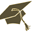Thesis (Selection of subject)(version: 285)
Assignment details
Login via CASAlgebraic proofs of Dirichlet's theorem on arithmetic progressions
Thesis title in Czech: Algebraické důkazy Dirichletovy věty o aritmetických posloupnostech Algebraic proofs of Dirichlet's theorem on arithmetic progressions Dirichletova věta, algebraická teorie čísel, prvočíslo, Chebotarevova věta o hustotě Dirichlet's theorem, algebraic number theory, primes, Chebotarev Density Theorem 2015/2016 Bachelor's thesis angličtina Department of Algebra (32-KA) Mgr. Vítězslav Kala, Ph.D. hidden- assigned and confirmed by the Study Dept. 13.10.2015 15.10.2015 07.12.2015 17.06.2016 00:00 26.05.2016 27.05.2016 17.06.2016 doc. Mgr. Pavel Příhoda, Ph.D.
 Guidelines Dirichlet's theorem says that there are infinitely many primes in every arithmetic progression ax+b with coprime a and b. The general proof is analytic, but in certain special cases it is possible to give more elementary, algebraic proofs. The goal of the thesis is to work out in detail the Euclidean proofs approach of  and solve related exercises. Besides from learning the basics of algebraic number theory this also requires non-trivial knowledge of Galois theory. The student may then possibly continue to study the generalization of this approach to arithmetic progressions in other number fields (involving applications of Tchebotarev density theorem).
 References  M. R. Murty, N. Thain. Prime numbers in certain arithmetic progressions: Funct. Approx. Comment. Math. 35 (2006), 249–259.  K. Conrad: Euclidean proofs of Dirichlet's theorem, www.math.uconn.edu/~kconrad/blurbs/dirichleteuclid.pdf.  J. S. Milne: Algebraic Number Theory, http://www.jmilne.org/math/CourseNotes/ant.html.  M. R. Murty, J. Esmonde: Problems in Algebraic Number Theory, GTM 190.  S. Lang: Algebraic Number Theory, GTM 110.

Charles University | Information system of Charles University | http://www.cuni.cz/UKEN-329.html